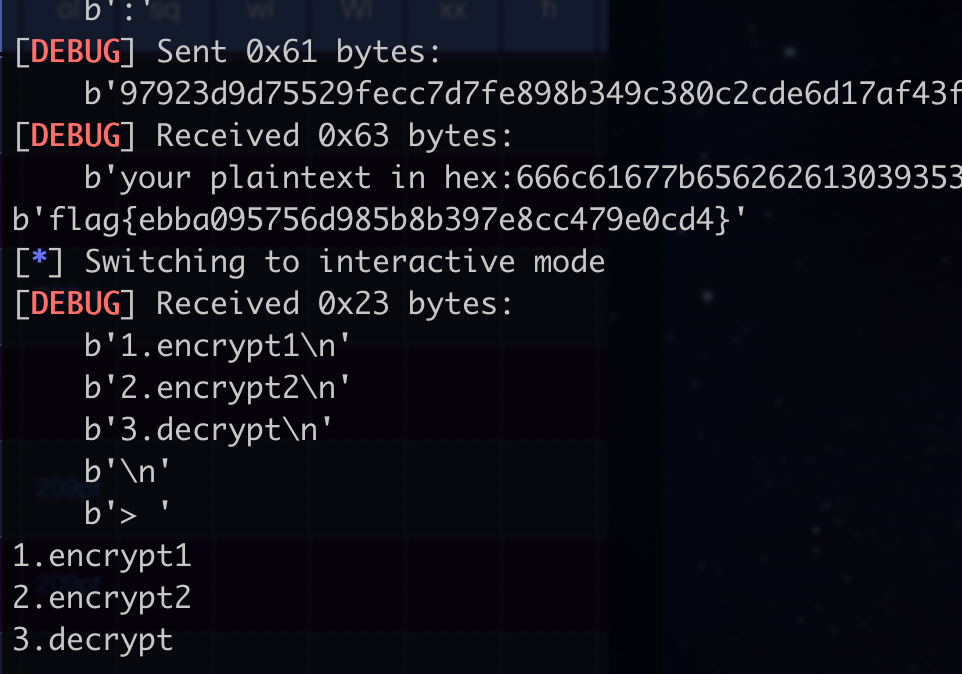# Case Study: Differetial Cryptanalysis Attack

## WMCTF idiot box

hellman yyds!

c’为第3个F函数input的差分值，D’为第4个F函数input的差分值，F’为第6个F函数output的差分值，$T_L'$是密文左半部分的差分值。

DES里面就sbox比较难搞，其他的部分就是一些线性置换，可以通过一些差分特性去操作一下这个sbox，然后就能得到key。

  1 2 3 4 5 6 7 8 9 10 11 12 13 14 15 16 17 18 19 20 21 22 23 24 25 26 27 28  from collections import Counter ... def gen_diff_output(diff): p1 = getRandomNBitInteger(32) p2 = p1 ^ diff k = getRandomNBitInteger(48) c1, c2 = F(p1, k), F(p2, k) return c1^c2, (p1,p2,c1,c2) counter = Counter() for i in range(10000): P_ = 0x00000040 X_, _ = gen_diff_output(P_) counter[X_] += 1 X_, freq = counter.most_common(1) print(hex(X_)[2:].rjust(8,'0'), freq / 10000) # 0x00000002 -> 0x00000002 0.217 # 0x00000040 -> 0x00000000 0.2534 # 0x00000400 -> 0x00000000 0.251 # 0x00000000 -> 0x00000000 1 # 0x00002000 -> 0x00000000 0.25 # 0x00004000 -> 0x00000040 0.22 # 0x00020000 -> 0x00020000 0.18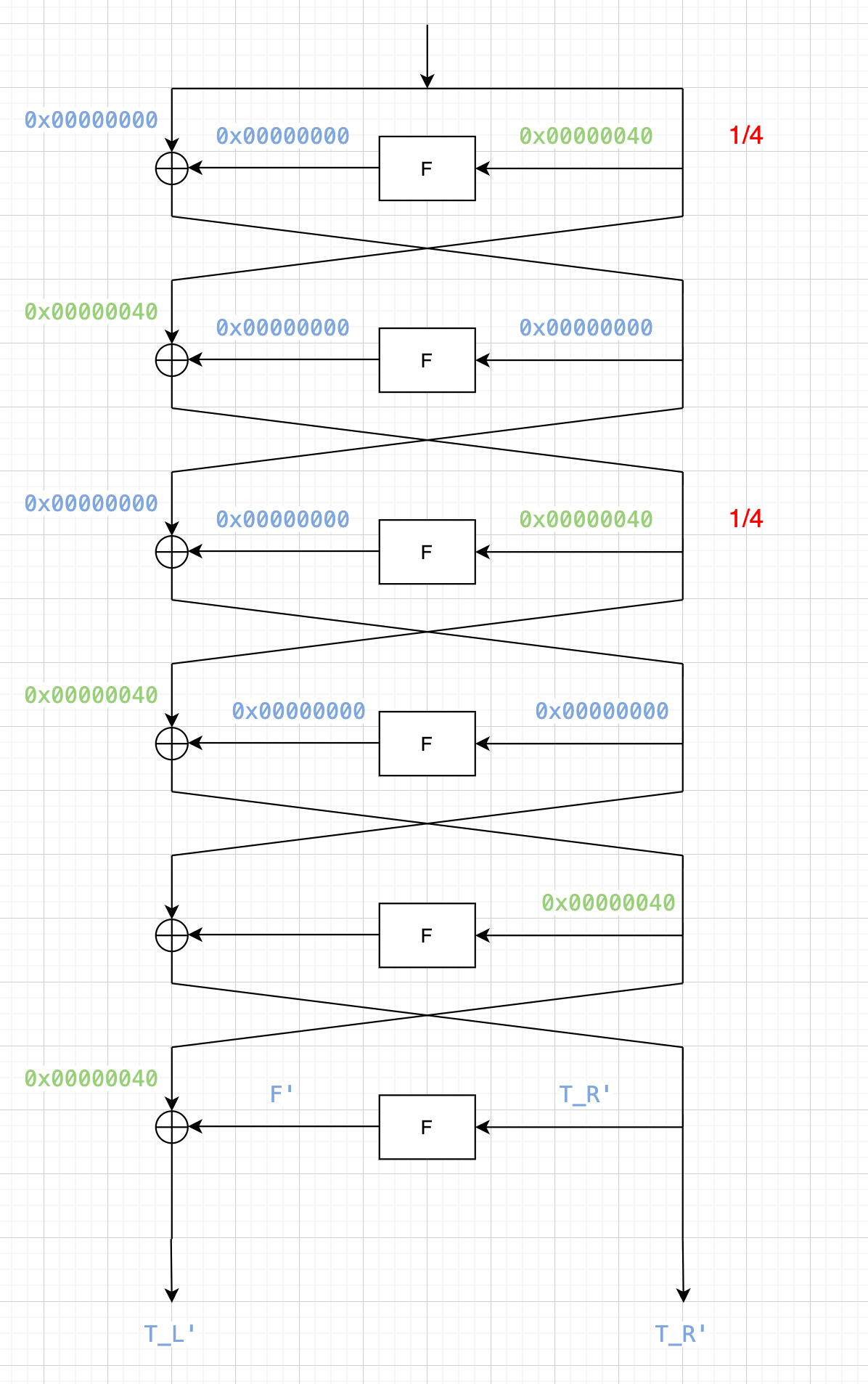1 2 3 4 5 6 7 8 9 10 11 12 13 14 15 16 17 18 19 20 21 22 23 24 25 26 27 28 29 30 31 32 33 34  import re from json import dump from tqdm import tqdm from Crypto.Util.number import long_to_bytes, getRandomNBitInteger from pwn import * def gen_diff_input(diff): p1 = getRandomNBitInteger(64) p2 = p1 ^ diff return p1, p2 r = remote("81.68.174.63", 34129) # context.log_level = "debug" rec = r.recvuntil(b"required").decode() cipher_flag = re.findall(r"\n([0-9a-f]{80})\n", rec) print(cipher_flag) r.recvline() pairs = [] for i in tqdm(range(10000)): p1, p2 = gen_diff_input(0x0000000000000040) r.sendline(long_to_bytes(p1).hex().encode()) c1 = int(r.recvline(keepends=False), 16) r.sendline(long_to_bytes(p2).hex().encode()) c2 = int(r.recvline(keepends=False), 16) pairs.append(((p1,p2), (c1,c2))) r.close() dump([cipher_flag, pairs], open("data", "w")) 

  1 2 3 4 5 6 7 8 9 10 11 12 13 14 15 16 17 18 19 20 21 22 23 24 25 26 27 28 29 30 31 32 33 34 35 36 37 38 39 40 41 42 43 44 45 46 47 48 49 50 51 52 53 54 55 56 57 58 59 60 61 62 63 64 65 66 67 68 69 70 71 72 73 74 75 76 77 78 79 80 81 82 83  from collections import Counter from json import load from tqdm import tqdm cipher_flag, pairs = load(open("data", "r")) ... def inv_key(key): inv_key = *48 key_bin = bin(key)[2:].rjust(48, '0') for j in range(48): inv_key[pc_key[j]] = key_bin[j] return int(''.join(inv_key), 2) def inv_keys(k6): keys = *6 keys[-1] = k6 for i in range(4,-1,-1): keys[i] = inv_key(keys[i+1]) return keys def inv_p(x): x_bin = [int(_) for _ in bin(x)[2:].rjust(32, '0')] y_bin = *32 for i in range(32): y_bin[pbox[i]] = x_bin[i] y = int(''.join([str(_) for _ in y_bin]), 2) return y # -------------------------- candidate_keys = [Counter() for _ in range(8)] for _, cs in tqdm(pairs): c1, c2 = cs if c1 ^ c2 == 0x0000004000000000: continue l1, l2 = c1 >> 32, c2 >> 32 r1, r2 = c1 & 0xffffffff, c2 & 0xffffffff # print(r1, r2) F_ = l1^l2^0x00000040 F_ = inv_p(F_) # xor of the two outputs of sbox, 32bit Ep1 = e(r1) # 48bit Ep2 = e(r2) # 48bit for i in range(8): inp1 = (Ep1 >> (7-i)*6) & 0b111111 # 6bit inp2 = (Ep2 >> (7-i)*6) & 0b111111 # 6bit out_xor = (F_ >> (7-i)*4) & 0b1111 # 4bit for key in range(64): if s(inp1^key, i) ^ s(inp2^key, i) == out_xor: candidate_keys[i][key] += 1 print(candidate_keys) # ---------------------- key6 = [] for c in candidate_keys: print(c.most_common(2)) key6.append(c.most_common(1)) print(key6) # key6 = [53, 44, 38, 7, 7, 30, 29, 52] k6 = sum(key6[i]<<(7-i)*6 for i in range(8)) # k6 = 236161043654516 keys = inv_keys(k6) print(keys) ps, cs = pairs p1, c1 = ps, cs assert enc_block(p1) == c1 # Ok! key is right! # To decrypt, reverse the keys. keys = keys[::-1] print(enc(bytes.fromhex(cipher_flag))) # b'WMCTF{D1ff3r3nti@1_w1th_1di0t_B0X3s}\x00\x00\x00\x00' 

WMCTF{D1ff3r3nti@1_w1th_1di0t_B0X3s}

## 强网杯2020 fault

differential fault attack SM4

Min WANG,Zhen WU,Jin-tao RAO,Hang LING. Round reduction-based fault attack on SM4 algorithm[J]. Journal on Communications, 2016, 37(Z1): 98-103.

We show that if a random byte fault is induced into either the second, third or fourth word register at the input of the 28-th round, the 128-bit master key could be derived with an exhaustive search of 22.11 bits on average.

28轮的第2、3、4个寄存器出错，可以直接整出master key，很对头

The procedure of the round-key generation indicates that the master key can be easily retrieved from any four consecutive round-keys.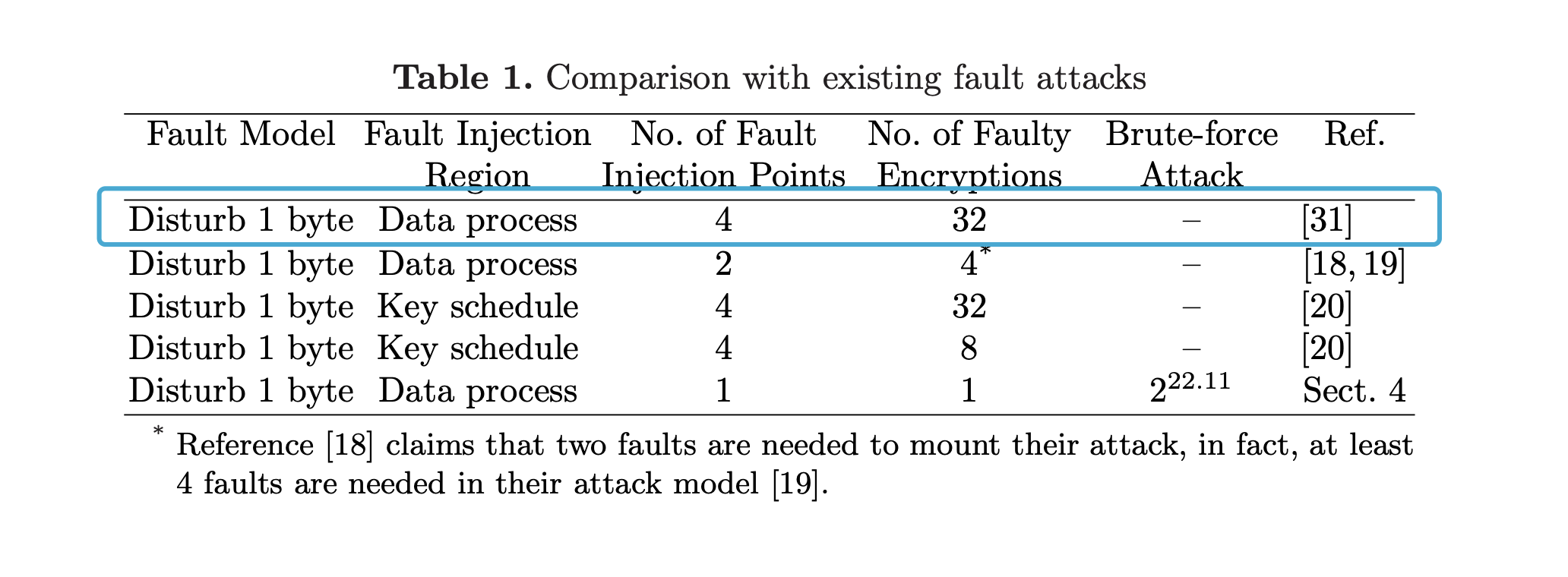paper：https://wenku.baidu.com/view/df86818e79563c1ec5da71c4.html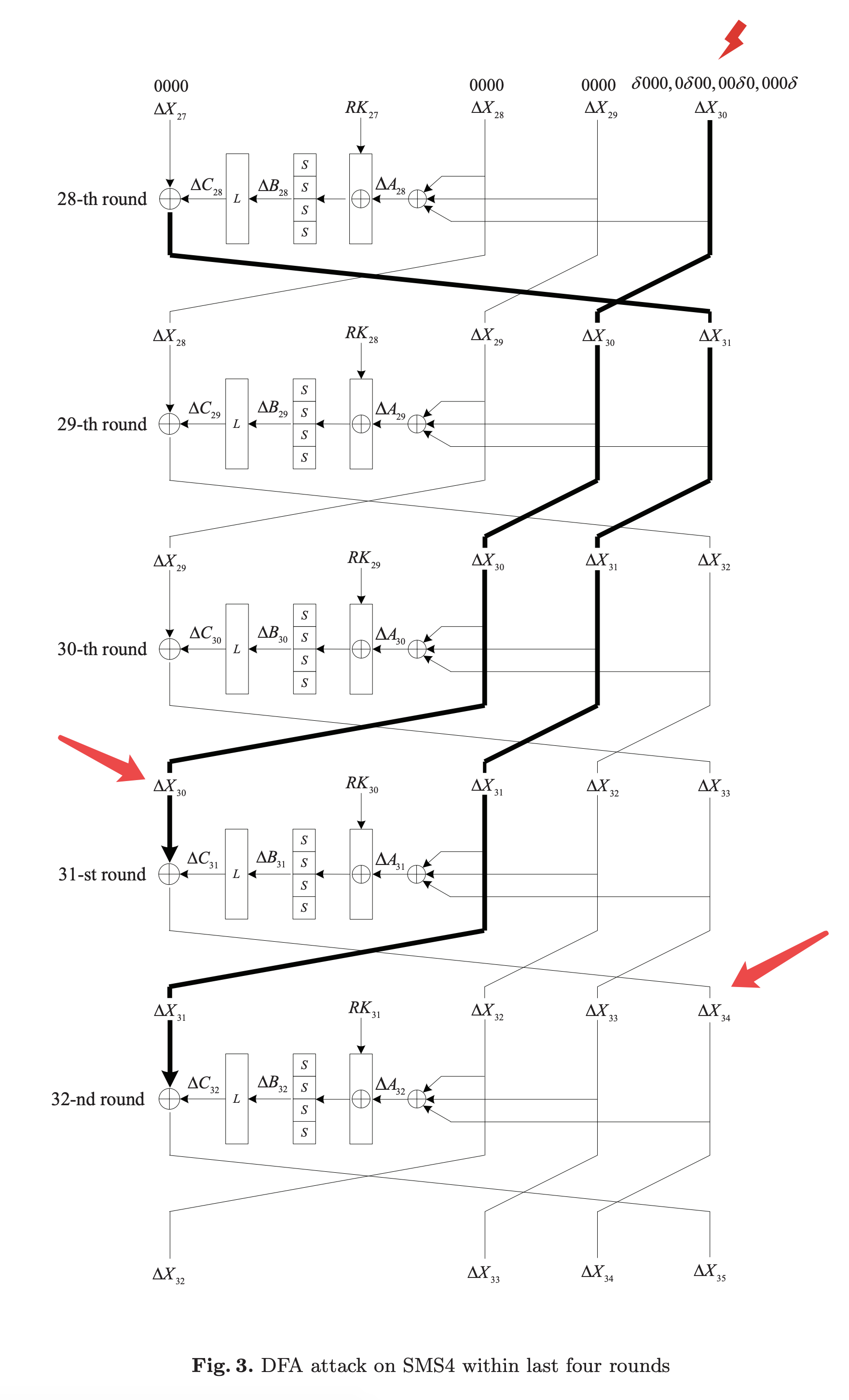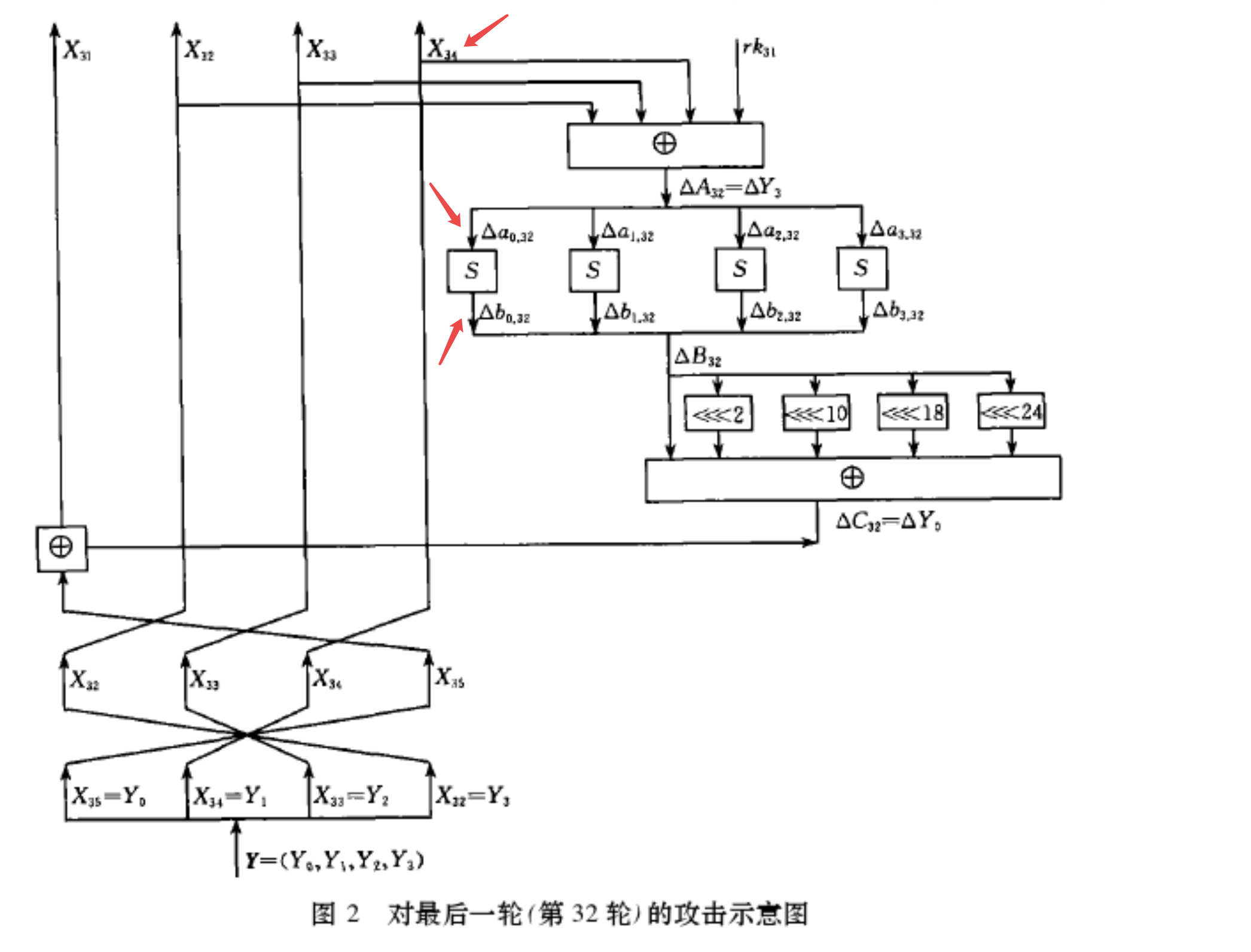r_byte: raw input byte f_byte: fault input byte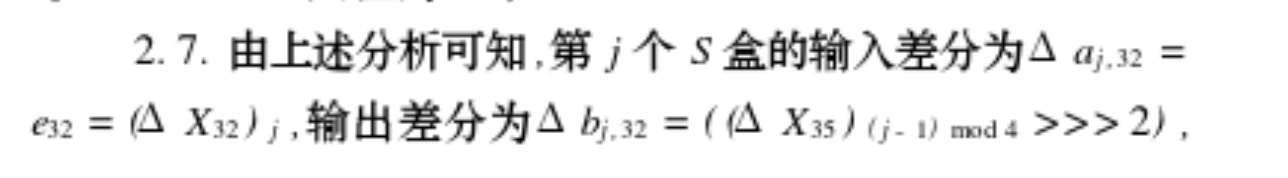paper里有具体的分析，看不懂，直接看到结论。这个结论就是说sbox输出的差分值diff_out也能确定下来。

ok，然后穷举这个sbox所对应那一byte子密钥rk_byte（仅256种可能，一个子密钥有4byte，每1byte对应一个sbox），计算sbox(r_inp ^ rk_byte) ^ sbox(f_inp ^ rk_byte)，看是否等于diff_out，如果等于就说明这个byte可以作为备选子密钥byte（理论值是说这边有2.0236个可能的子密钥byte）。两次这么操作后，基本上就可以确定下这个byte到底是哪一个了。

key schedule可逆，能直接搞到master key

  1 2 3 4 5 6 7 8 9 10 11 12 13 14 15 16 17 18 19 20 21 22 23 24 25 26 27 28 29 30 31 32 33 34 35 36 37 38 39 40 41 42 43 44 45 46 47 48 49 50 51 52 53 54 55 56 57 58 59 60 61 62 63 64 65 66 67 68 69 70 71 72 73 74 75 76 77 78 79 80 81 82 83 84 85 86 87 88 89 90 91 92 93 94 95 96 97 98 99 100 101 102 103 104 105 106 107 108 109 110 111 112 113 114 115 116 117 118 119 120 121 122 123 124 125 126 127 128 129 130 131 132 133 134 135 136 137 138 139 140 141 142 143 144 145 146 147 148 149 150 151 152 153 154 155 156 157 158 159 160 161 162 163 164 165 166 167 168 169 170 171 172 173 174 175 176 177 178 179 180 181 182 183 184 185 186 187 188 189 190 191 192 193 194 195 196 197 198 199 200 201  from collections import Counter import random from itertools import product from hashlib import sha256 from pwn import * from sm4 import * from func import xor, rotl, get_uint32_be, put_uint32_be, \ bytes_to_list, list_to_bytes, padding, unpadding token = b"icq3f18237ca27013a7969864ab40836" r = remote("39.101.134.52", 8006) # context.log_level = 'debug' # PoW rec = r.recvline().decode() suffix = re.findall(r'XXX\+([^\)]+)', rec) digest = re.findall(r'== ([^\n]+)', rec) print(f"suffix: {suffix} \ndigest: {digest}") print('Calculating hash...') for i in product(string.ascii_letters + string.digits, repeat=3): prefix = ''.join(i) guess = prefix + suffix if sha256(guess.encode()).hexdigest() == digest: print(guess) break r.sendafter(b'Give me XXX:', prefix.encode()) r.sendafter(b"teamtoken", token) r.recvuntil(b"your flag is\n") enc_flag = r.recvline().strip() print(enc_flag) plaintext = b"\x00" * 15 def ltor(b, l): bits = bin(b)[2:] return int(bits[-l:] + bits[:-l], 2) def inv_Y(cipher): # bytes -> list Y0 = get_uint32_be(cipher[0:4]) Y1 = get_uint32_be(cipher[4:8]) Y2 = get_uint32_be(cipher[8:12]) Y3 = get_uint32_be(cipher[12:16]) # X32, X33, X34, X35 return [Y3, Y2, Y1, Y0] def inv_round(Xs): return [Xs[-1], Xs, Xs, Xs] def get_rk_byte(raw_cipher, fault_ciphers, j): r_res, r_X32, r_X33, r_X34 = inv_round(raw_cipher) r_byte = put_uint32_be(r_X32 ^ r_X33 ^ r_X34)[j%4] ios = [] for f_cipher in fault_ciphers: f_res, f_X32, f_X33, f_X34 = inv_round(f_cipher) diff_out = ltor(put_uint32_be(r_res ^ f_res)[(j-1)%4], 2) f_byte = put_uint32_be(f_X32 ^ f_X33 ^ f_X34)[j%4] ios.append((f_byte,diff_out)) # print(ios) candidate_keys = Counter() for rk_byte in range(256): for f_byte, diff_out in ios: if SM4_BOXES_TABLE[r_byte^rk_byte] ^ SM4_BOXES_TABLE[f_byte^rk_byte] == diff_out: candidate_keys[rk_byte] += 1 return candidate_keys.most_common() def get_r_cipher(): r.sendlineafter(b"> ", b"1") r.sendlineafter(b"your plaintext in hex:", plaintext.hex().encode()) cipher = bytes.fromhex(r.recvline().strip().decode().split("hex:")) return cipher def get_f_cipher(round, j): r.sendlineafter(b"> ", b"2") r.sendlineafter(b"your plaintext in hex:", plaintext.hex().encode()) r.sendlineafter(b"give me the value of r f p:", f"{round} {random.getrandbits(8)} {j}") cipher = bytes.fromhex(r.recvline().strip().decode().split("hex:")) return cipher def f(x0, x1, x2, x3, rk): # "T algorithm" == "L algorithm" + "t algorithm". # args: [in] a: a is a 32 bits unsigned value; # return: c: c is calculated with line algorithm "L" and nonline algorithm "t" def _sm4_l_t(ka): b = [0, 0, 0, 0] a = put_uint32_be(ka) b = SM4_BOXES_TABLE[a] b = SM4_BOXES_TABLE[a] b = SM4_BOXES_TABLE[a] b = SM4_BOXES_TABLE[a] bb = get_uint32_be(b[0:4]) c = bb ^ (rotl(bb, 2)) ^ (rotl(bb, 10)) ^ (rotl(bb, 18)) ^ (rotl(bb, 24)) return c return (x0 ^ _sm4_l_t(x1 ^ x2 ^ x3 ^ rk)) def decrypt_one_round(cipher, rk): return [f(cipher, cipher, cipher, cipher, rk), cipher, cipher, cipher] def decrypt_rounds(cipher, rks): for rk in rks: cipher = decrypt_one_round(cipher, rk) return cipher raw_cipher = inv_Y(get_r_cipher()) print(raw_cipher) rks = [] for round in range(31, 27, -1): # print(round) rk = 0 for j in range(4): fault_ciphers = set() for k in range(10): fault_ciphers.add(get_f_cipher(round, j)) fault_ciphers = [inv_Y(i) for i in fault_ciphers] fault_ciphers = [decrypt_rounds(f_cipher, rks) for f_cipher in fault_ciphers] rk_byte = get_rk_byte(raw_cipher, fault_ciphers, j) rk = (rk << 8) + rk_byte print(f"round {round+1} subkey: {rk}") rks.append(rk) raw_cipher = decrypt_one_round(raw_cipher, rk) def _round_key(ka): b = [0, 0, 0, 0] a = put_uint32_be(ka) b = SM4_BOXES_TABLE[a] b = SM4_BOXES_TABLE[a] b = SM4_BOXES_TABLE[a] b = SM4_BOXES_TABLE[a] bb = get_uint32_be(b[0:4]) rk = bb ^ (rotl(bb, 13)) ^ (rotl(bb, 23)) return rk # def set_key(key, mode): # key = bytes_to_list(key) # sk = []*32 # MK = [123, 456, 789, 145] # k = *36 # MK = get_uint32_be(key[0:4]) # MK = get_uint32_be(key[4:8]) # MK = get_uint32_be(key[8:12]) # MK = get_uint32_be(key[12:16]) # k[0:4] = xor(MK[0:4], SM4_FK[0:4]) # for i in range(32): # k[i + 4] = k[i] ^ ( # _round_key(k[i + 1] ^ k[i + 2] ^ k[i + 3] ^ SM4_CK[i])) # sk[i] = k[i + 4] # return sk def inv_key_schedule(rks): k =  * 32 + rks[::-1] for i in range(31, -1, -1): k[i] = k[i+4] ^ (_round_key(k[i + 1] ^ k[i + 2] ^ k[i + 3] ^ SM4_CK[i])) print(k[4:]) Mk =  * 4 for j in range(4): Mk[j] = SM4_FK[j] ^ k[j] master_key = [] for i in range(4): master_key += put_uint32_be(Mk[i]) return list_to_bytes(master_key) Mk = inv_key_schedule(rks) print(Mk) r.sendlineafter(b"> ", b"3") r.sendlineafter(b"your key in hex:", Mk.hex().encode()) r.sendlineafter(b"your ciphertext in hex:", enc_flag) r.recvuntil(b"your plaintext in hex:") flag = r.recvline().strip().decode() print(bytes.fromhex(flag)) r.interactive()